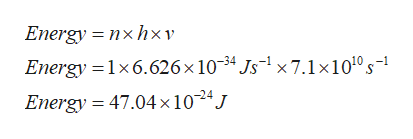The frequency of a Microwave wave emitted from an unknown source is measured and found to be 7.1 x 1010 Hz. What is the energy in Joules of one quanta of this radiation ? 1 Hz = 1 s-1Enter your answer here: J

Question

The frequency of a Microwave wave emitted from an unknown source is measured and found to be 7.1 x 1010 Hz. What is the energy in Joules of one quanta of this radiation ? 1 Hz = 1 s-1

Step 1

Energy of radiation is given by formula:

E=nhv where n=no. of quanta=1

h= planck\\'s constant =6.626×10-34  Js-1

v= frequency of radiatio...help_outlineImage TranscriptioncloseEnergy nxhxv Energy 1x6.626 x 10-34 Js1x7.1x1010 s- Energy = 47.04x 1024J fullscreen

Want to see the full answer?

See Solution

Want to see this answer and more?

Our solutions are written by experts, many with advanced degrees, and available 24/7

See Solution
Tagged in

Atomic structure